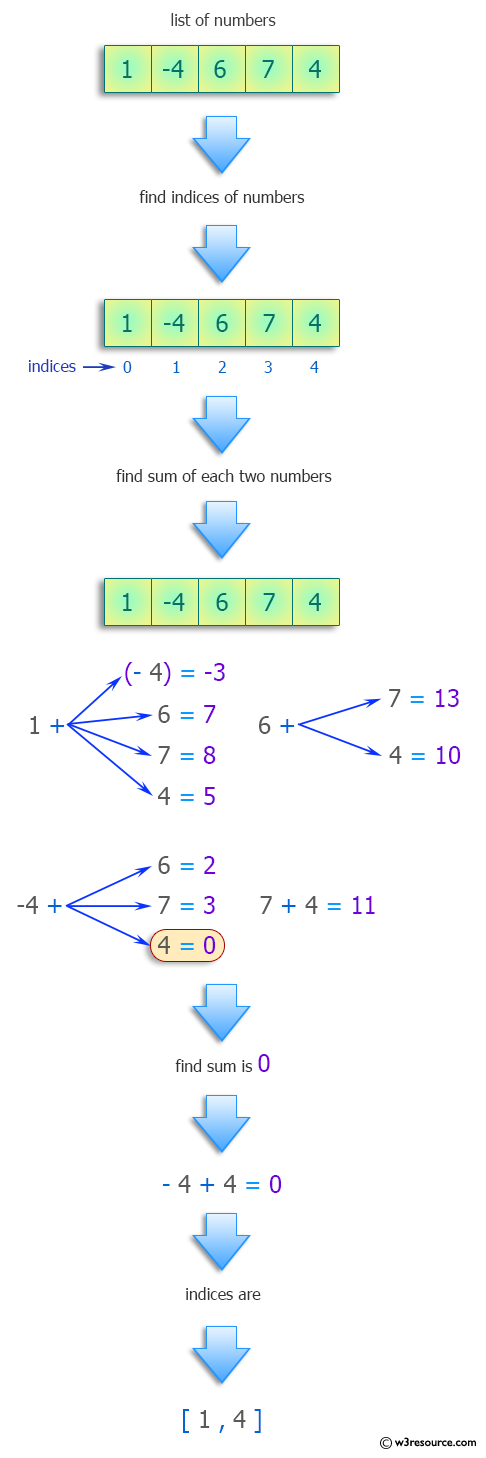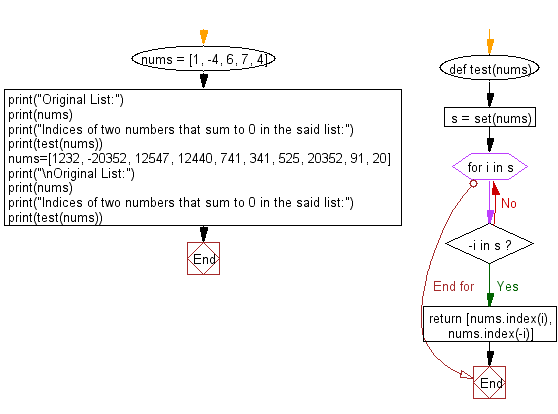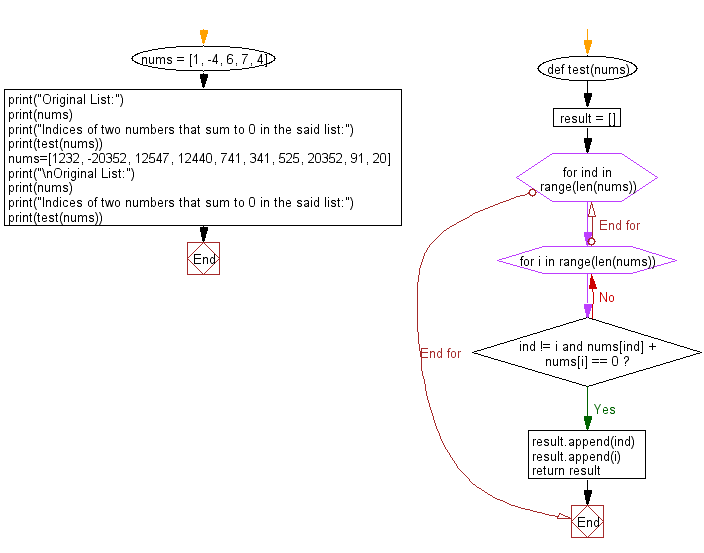﻿ Python: Find the indices of two numbers that sum to 0 in a given list - w3resource# Python: Find the indices of two numbers that sum to 0 in a given list

## Python Programming Puzzles: Exercise-29 with Solution

Write a Python program to find the indices of two numbers that sum to 0 in a given list of numbers.

```Input:
[1, -4, 6, 7, 4]
Output:
[4, 1]

Input:
[1232, -20352, 12547, 12440, 741, 341, 525, 20352, 91, 20]
Output:
[1, 7]
```

Pictorial Presentation:Sample Solution-1:

Python Code:

``````#License: https://bit.ly/3oLErEI

def test(nums):
s = set(nums)
for i in s:
if -i in s:
return [nums.index(i), nums.index(-i)]

nums = [1, -4, 6, 7, 4]
print("Original List:")
print(nums)
print("Indices of two numbers that sum to 0 in the said list:")
print(test(nums))
nums=[1232, -20352, 12547, 12440, 741, 341, 525, 20352, 91, 20]
print("\nOriginal List:")
print(nums)
print("Indices of two numbers that sum to 0 in the said list:")
print(test(nums))
``````

Sample Output:

```Original List:
[1, -4, 6, 7, 4]
Indices of two numbers that sum to 0 in the said list:
[4, 1]

Original List:
[1232, -20352, 12547, 12440, 741, 341, 525, 20352, 91, 20]
Indices of two numbers that sum to 0 in the said list:
[1, 7]
```

Flowchart:## Visualize Python code execution:

The following tool visualize what the computer is doing step-by-step as it executes the said program:

Sample Solution-2:

Python Code:

``````#License: https://bit.ly/3oLErEI

def test(nums):
result = []
# For each index in the list:
for ind in range(len(nums)):
# For each index in the list:
for i in range(len(nums)):
# If the number at the current index and the other index sum to zero
if ind != i and nums[ind] + nums[i] == 0:
result.append(ind)
result.append(i)
# Found the indices; no need to go through the whole list
return result

nums = [1, -4, 6, 7, 4]
print("Original List:")
print(nums)
print("Indices of two numbers that sum to 0 in the said list:")
print(test(nums))
nums=[1232, -20352, 12547, 12440, 741, 341, 525, 20352, 91, 20]
print("\nOriginal List:")
print(nums)
print("Indices of two numbers that sum to 0 in the said list:")
print(test(nums))
``````

Sample Output:

```Original List:
[1, -4, 6, 7, 4]
Indices of two numbers that sum to 0 in the said list:
[1, 4]

Original List:
[1232, -20352, 12547, 12440, 741, 341, 525, 20352, 91, 20]
Indices of two numbers that sum to 0 in the said list:
[1, 7]
```

Flowchart:## Visualize Python code execution:

The following tool visualize what the computer is doing step-by-step as it executes the said program:

Python Code Editor :

Have another way to solve this solution? Contribute your code (and comments) through Disqus.

What is the difficulty level of this exercise?

Test your Programming skills with w3resource's quiz.

﻿

## Python: Tips of the Day

Find the index of an item in a list?

Given a list ["foo", "bar", "baz"] and an item in the list "bar", how do I get its index (1) in Python?

```>>> ["foo", "bar", "baz"].index("bar")
1
```

Caveats follow

Note that while this is perhaps the cleanest way to answer the question as asked, index is a rather weak component of the list API, and I can't remember the last time I used it in anger. It's been pointed out to me in the comments that because this answer is heavily referenced, it should be made more complete. Some caveats about list.index follow. It is probably worth initially taking a look at the documentation for it:

```list.index(x[, start[, end]])
```

Linear time-complexity in list length

An index call checks every element of the list in order, until it finds a match. If your list is long, and you don't know roughly where in the list it occurs, this search could become a bottleneck. In that case, you should consider a different data structure. Note that if you know roughly where to find the match, you can give index a hint. For instance, in this snippet, l.index(999_999, 999_990, 1_000_000) is roughly five orders of magnitude faster than straight l.index(999_999), because the former only has to search 10 entries, while the latter searches a million:

```>>> import timeit
>>> timeit.timeit('l.index(999_999)', setup='l = list(range(0, 1_000_000))', number=1000)
9.356267921015387
>>> timeit.timeit('l.index(999_999, 999_990, 1_000_000)', setup='l = list(range(0, 1_000_000))', number=1000)
0.0004404920036904514
```

Only returns the index of the first match to its argument

A call to index searches through the list in order until it finds a match, and stops there. If you expect to need indices of more matches, you should use a list comprehension, or generator expression.

```>>> [1, 1].index(1)
0
>>> [i for i, e in enumerate([1, 2, 1]) if e == 1]
[0, 2]
>>> g = (i for i, e in enumerate([1, 2, 1]) if e == 1)
>>> next(g)
0
>>> next(g)
2
```

Most places where I once would have used index, I now use a list comprehension or generator expression because they're more generalizable. So if you're considering reaching for index, take a look at these excellent Python features.

Throws if element not present in list

A call to index results in a ValueError if the item's not present.

```>>> [1, 1].index(2)
Traceback (most recent call last):
File "<stdin>", line 1, in <module>
ValueError: 2 is not in list
```

If the item might not be present in the list, you should either

• Check for it first with item in my_list (clean, readable approach), or
• Wrap the index call in a try/except block which catches ValueError (probably faster, at least when the list to search is long, and the item is usually present.)

Ref: https://bit.ly/2ALwXwe